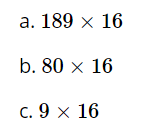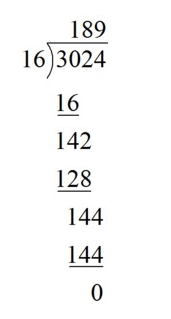Match Fishtank is now Fishtank Learning!

# Multi-Digit and Fraction Computation

## Objective

Divide multi-digit whole numbers using the standard algorithm.

## Common Core Standards

### Core Standards

?

• 6.NS.B.2 — Fluently divide multi-digit numbers using the standard algorithm.

?

• 4.NBT.B.6

• 5.NBT.B.6

## Criteria for Success

?

1. Divide multi-digit whole numbers using partial quotients.
2. Understand the connection between the standard algorithm for division and partial quotients.
3. Apply the standard algorithm for division to solve problems.

## Tips for Teachers

?

• In fifth grade, students found whole number quotients with up to 2 digit divisors and 4 digit dividends using various strategies. In sixth grade, dividing multi-digit whole numbers is a fluency expectation. This lesson reviews partial quotient strategies from fifth grade and students are guided toward understanding the standard algorithm as an efficient way to compute. Ensure students have opportunities to build towards fluency throughout the unit and the year. See our Guide to Procedural Skill and Fluency for additional information and strategies.
• The following tools or materials are useful for this lesson: large graph or grid paper for computations, calculators to check answers.

#### Remote Learning Guidance

If you need to adapt or shorten this lesson for remote learning, we suggest prioritizing Anchor Problem 2 or 3 (benefit from worked examples). If students have a strong grasp on using the standard algorithm to divide whole numbers, then this Anchor Problem can either be skipped or done independently. Find more guidance on adapting our math curriculum for remote learning here.

#### Fishtank Plus

• Problem Set
• Student Handout Editor
• Vocabulary Package

## Anchor Problems

?

### Problem 1

Find the quotient of ${ 67,764 \div 12}$ using partial quotients.

### Problem 2

Solve ${70,072 \div 19}$ using the standard algorithm.

#### References

EngageNY Mathematics Grade 6 Mathematics > Module 2 > Topic C > Lesson 13Example 1

Grade 6 Mathematics > Module 2 > Topic C > Lesson 13 of the New York State Common Core Mathematics Curriculum from EngageNY and Great Minds. © 2015 Great Minds. Licensed by EngageNY of the New York State Education Department under the CC BY-NC-SA 3.0 US license. Accessed Dec. 2, 2016, 5:15 p.m..

Modified by Fishtank Learning, Inc.

### Problem 3

Solve ${48,072 \div 24}$ using the standard algorithm.

### Problem 4

Use the computation shown to find the products.#### References

Illustrative Mathematics Interpreting a Division Computation

Interpreting a Division Computation, accessed on Sept. 28, 2017, 3:31 p.m., is licensed by Illustrative Mathematics under either the CC BY 4.0 or CC BY-NC-SA 4.0. For further information, contact Illustrative Mathematics.

## Problem Set

?The following resources include problems and activities aligned to the objective of the lesson that can be used to create your own problem set.

• Include a few problems where there is a remainder. (Students are familiar with remainders from fourth and fifth grades.)